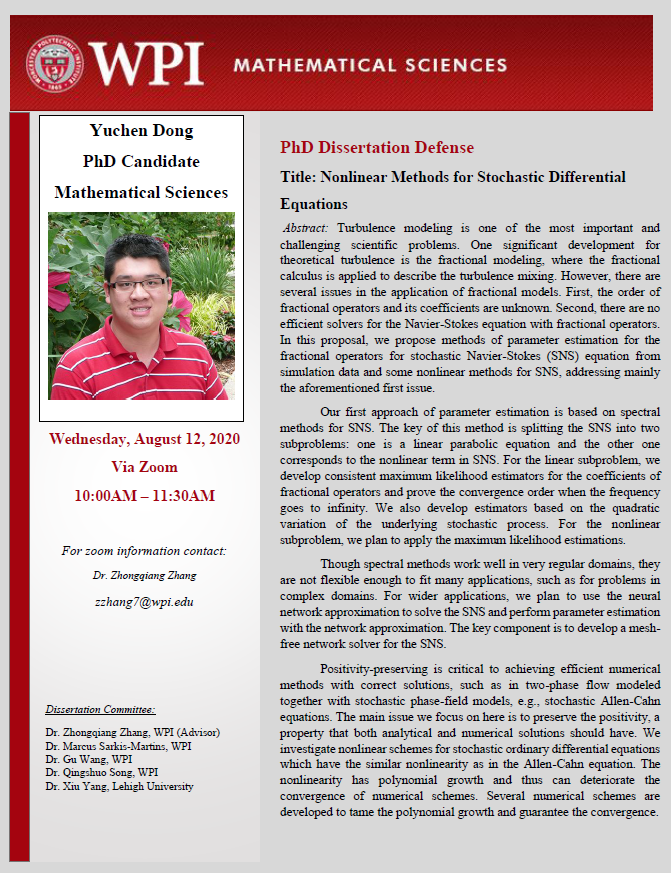# Mathematical Sciences-PhD Dissertation Defense-Yuchen Dong -"Nonlinear Numerical Methods for Stochastic Differential Equations"

## YuchenDongflyer.pngWednesday, August 12, 2020
10:00 am to 11:30 am

PhD Dissertation Defense
Title: Nonlinear Methods for Stochastic Differential Equations
PhD Candidate: Yuchen Dong

Abstract: Turbulence modeling is one of the most important and challenging scientific problems. One significant development for theoretical turbulence is the fractional modeling, where the fractional calculus is applied to describe the turbulence mixing. However, there are several issues in the application of fractional models. First, the order of fractional operators and its coefficients are unknown. Second, there are no efficient solvers for the Navier-Stokes equation with fractional operators. In this proposal, we propose methods of parameter estimation for the fractional operators for stochastic Navier-Stokes (SNS) equation from simulation data and some nonlinear methods for SNS, addressing mainly the aforementioned first issue.

Our first approach of parameter estimation is based on spectral methods for SNS. The key of this method is splitting the SNS into two subproblems: one is a linear parabolic equation and the other one corresponds to the nonlinear term in SNS. For the linear subproblem, we develop consistent maximum likelihood estimators for the coefficients of fractional operators and prove the convergence order when the frequency goes to infinity. We also develop estimators based on the quadratic variation of the underlying stochastic process. For the nonlinear subproblem, we plan to apply the maximum likelihood estimations.

Though spectral methods work well in very regular domains, they are not flexible enough to fit many applications, such as for problems in complex domains. For wider applications, we plan to use the neural network approximation to solve the SNS and perform parameter estimation with the network approximation. The key component is to develop a mesh-free network solver for the SNS.

Positivity-preserving is critical to achieving efficient numerical methods with correct solutions, such as in two-phase flow modeled together with stochastic phase-field models, e.g., stochastic Allen-Cahn equations. The main issue we focus on here is to preserve the positivity, a property that both analytical and numerical solutions should have. We investigate nonlinear schemes for stochastic ordinary differential equations which have the similar nonlinearity as in the Allen-Cahn equation. The nonlinearity has polynomial growth and thus can deteriorate the convergence of numerical schemes. Several numerical schemes are developed to tame the polynomial growth and guarantee the convergence.

Dissertation Committee: